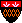Introduction to Automata Theory, Languages, and Computation: Errata for First and Second Printings Only
List of Errata for the First Printing Only.

In addition: the following were not corrected in time for the second printing, but were corrected in the third printing:

p. 17, l. 4: "H and C" should be "H and not C" (Thanks to Mark Meuleman).

p. 20, l. -14: "it" should be capitalized (Thanks to Mark Meuleman).

p. 78, l. 2: delta should be delta_E (Thanks to Geoff Johnstone).

p. 91, 4 lines above Th. 3.4: "indictively" -> "inductively" (Thanks to Ben Pfaff).

p. 120, l. 10: hyphen in "Only if"; similar mistakes appear on pp. 178, 402, 406, 441, 442, 451, 453, 457, and 472 (Thanks to Ben Pfaff).

p. 137, l. 1: "seen only 1's" (not "0's") (Thanks to ThriBhuvan Singh).

p. 174, l. -19: "identifier" -> "expression" (Thanks to Rod Topor).

p. 174, l. -8: quotes after "steps" (Thanks to Christian Lemburg).

p. 193, l. -4: The last production body should be iSeS (Thanks to Rod Topor).

p. 204, Exercise 5.3.3, l. 2: The last production body should be iSeS (Thanks to Rod Topor).

p. 225, l. 3: "turnstyle" -> "turnstile" (Thanks to Christian Lemburg).

p. 233, l. 1 of footnote: The new states are p and r (Thanks to Jacinth H.T. Wu).

p. 236, l. -4: "and" -> "an" (Thanks to Rod Topor).

p. 238, l. -9: P should be G (Thanks to Rod Topor).

p. 250, l. 15: "by" -> "be" (Thanks to Rod Topor).

p. 271, Exercise 7.1.2: It is safer to perform the useless-symbol elimination after eliminating epsilon-productions and unit productions. Thus, we should have asked for the steps in the order (b), (c), (a), (d). The same applies to Exercises 7.1.3 - 7.1.5.

p. 320, last line: The following is an equivalent, but clearer way to express the move: qX_1X_2...X_n |- pBYX_2...X_n (Thanks to Robert Thomson).

p. 321, l. 13: The X_{n-1} at the end should be X_n (Thanks to Robert Thomson).

p. 324, item 1, l. 2: m+1 -> n+1 (Thanks to Dmitry Shkatov).

p. 326, l. 1: B -> 1 (Thanks to Dmitry Shkatov).

p. 327, l. -9: "the" needed in front of "language" (Thanks to Mark Meuleman).

p. 331, bottom: The second component of the state can also be the blank (Thanks to Mike Lederle).

p. 339, l. -19: "we" should be capitalized (Thanks to Mark Meuleman).

p. 340, l. 10: "Thus if M starts" -> "Thus if N starts" (Thanks to Jacinth H.T. Wu).

p. 340, l. -6: "a a" -> "a" (Thanks to Jacinth H.T. Wu).

p. 354, l. 8: "and odd" -> "an odd" (Thanks to Jacinth H.T. Wu).

p. 369, first two bullets: We need to use some other variables, such as r and s for the numbers of states and tape symbols, because they should not be confused with the indexes k and m used in the last paragraph on p. 369, which are particular states and symbols.

p. 371, l. 1: delete one "consecutive."

p. 375, l. 2: "to with" -> "to do with" (Thanks to Kang-Rae Lee).

p. 391, l. 12: "let" -> "Let" (Thanks to Mustafa Sait-Ametov).

p. 397, l. 19: i_{k+1} -> k+1 (Thanks to Mustafa Sait-Ametov).

p. 397, l. 23: z_{k_1} -> z_{k+1} (Thanks to Mustafa Sait-Ametov).

p. 399, l. -2: a_3 -> q_3 (Thanks to Mustafa Sait-Ametov).

p. 408, l. -3: The argument for (f) is the "same as (e)," not "same as (f)" (Thanks to Jacinth H.T. Wu).

p. 421, l. -4: P_1 and P_2 are interchanged (Thanks to Jacinth H.T. Wu).

p. 432, last line: parentheses around the entire line are needed (Thanks to Zeki Bayram).

p. 436, footnote: "Conjunction" is actually a fancy term for logical AND, not OR (Thanks to John Rappaport).

p. 438, l. -8: the middle "and" should be "an" (Thanks to Jacinth H.T. Wu).

p. 441, l. -18: delete "an" (Thanks to Ben Pfaff).

p. 442, l. 12 and 29: "If" and "Only if" labels on the proof parts are interchanged (Thanks to Jacinth H.T. Wu).

p. 442, l. 21-22: We must add to item (3) the condition that if T(x) is defined, then we must choose S(x) = T(x) (Thanks to Zeki Bayram).

p. 450, last line of box: delete "a" before "factor" (Thanks to Ben Pfaff).

p. 465, l. 1-3: The edge-cover problem is actually polynomial; it is a special case of 2SAT. Here is a similar substitute problem that is NP-complete: The feedback edge problem: given a graph G and an integer k, does there exist a set of k edges such that every cycle of G has at least one edge in the cycle? (Thanks to Richard Lorentz).

p. 467, last line: "10.4.4(d)" should be "10.4.4(g)" (Thanks to Junghoo Cho).

p. 468, ref. 2: Period needed in "A. Cobham" (Thanks to Kang-Rae Lee).

p. 472, l. 21: "L is in NP" should be "L-bar (i.e., L with a line above) is in NP" (Thanks to Jacinth H.T. Wu).

p. 477, l. -7: Omit "log_2" from the formula for m (Thanks to Zeki Bayram).

p. 491, l. 16: delete "are" (Thanks to Zeki Bayram).

p. 493, item (3), line 1: p(n) -> T(n) (Thanks to Zeki Bayram).

p. 494, l. 16: "none of" -> "at least one of" (Thanks to Zeki Bayram).

p. 501, l. 14: "throwing away" -> "then computing" (Thanks to Zeki Bayram).

p. 502, l. 17: add "modulo p" at the end (Thanks to Zeki Bayram).

p. 510, ref. 4: The date, 1972, is missing (Thanks to Zeki Bayram).